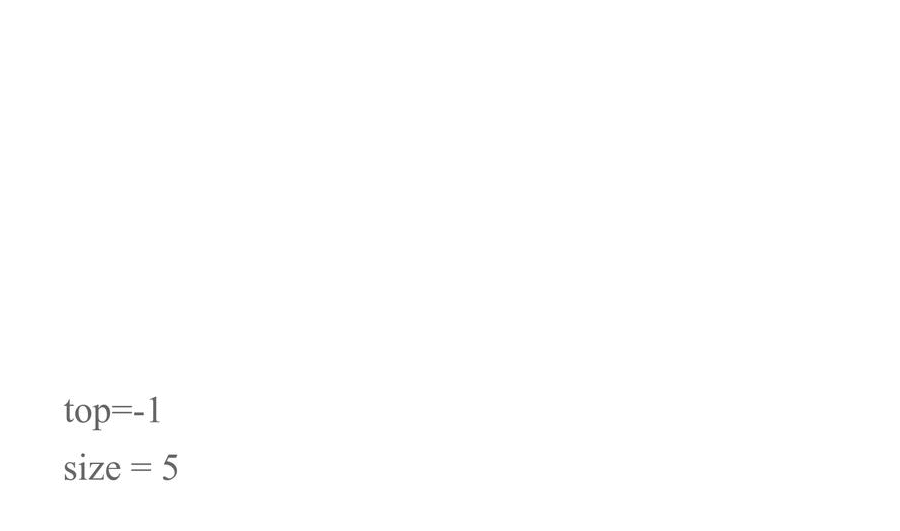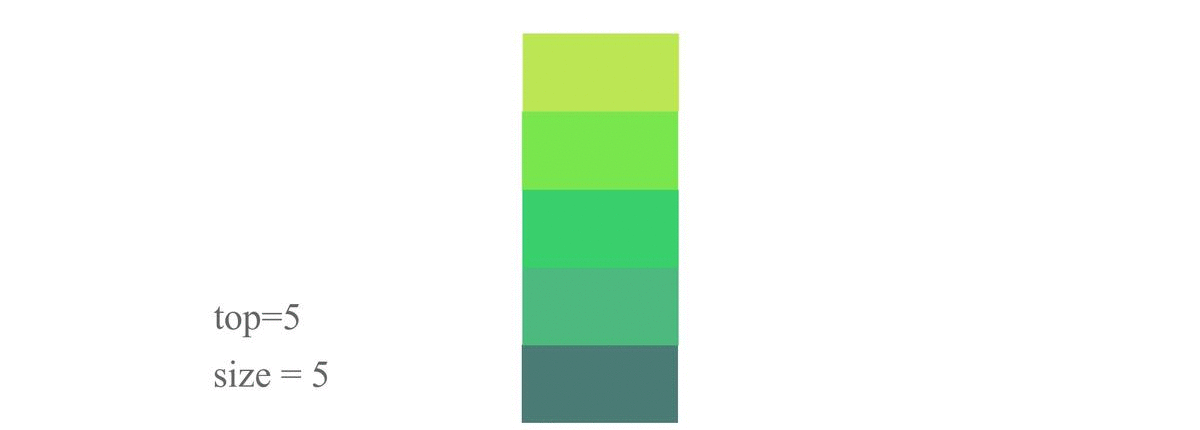Created July 4, 2021

So let me give you a quick recap, a linked list is a dynamic linear data structure. For making a linked list we can allocate blocks of memory located in different parts of the memory of the computer. So its basically a list but it's elements, instead of being located all at one place, they're situated randomly in the memory unlike an array which stores all its elements at the same place and is not dynamic.

So Linked list is just a data structure like an array and it can be used to implement concepts like stacks and queues. We've already seen the array implementation of the stacks. If you haven't yet, please it check it out here.

## Basic definition of Stack

Here we're gonna define the data type for stack as well as the linked list and both will be merged.

First we'll define Linked List and then use it in the stack data type.

``````typedef struct node{
int data;
struct node *next;
}NODE;
typedef struct st{
int size;
int top;
NODE *elements;
}STACK;
``````

## Push

Here we'll be implementing the insertion operation on the stack otherwise called the Push Operation. So first we make a node containing the user entered integer value. Then that new node will be linked to the stack's linked list. Steps:

1. Createnode function which creates node containing the number entered by the user. You can learn more about it here
2. Check if the top is equal to size. If it is then print "Overflow". Else do this -
3. Check if the stack's linked list is empty or not. If it is then set the head node equal to the new node. If not then do the following: 1. Iterate to the end of the list. 2. Set the next of the last node equal to the new node. 3. Increase the top value by 1. Code:
``````NODE *createnode(int data){
NODE *newnode = (NODE *)malloc(sizeof(NODE));
newnode->data = data;
newnode->next = NULL;
return newnode;
}
void push(int val, STACK *s){
NODE *newnode = createnode(val);
if(s->elements == NULL){//check if the stack is empty or not;
s->elements = newnode;
s->top=0;
}
else{
if(s->size-1 == s->top){//now check for the overflow;
printf("STACK OVERFLOW!\n");
}else{
s->top++;
NODE *temp = s->elements;//else iterate to the end and insert the node;
while(temp->next!=NULL){
temp = temp->next;
}//temp reaches the end;
temp->next = newnode;
}
}
}
``````## Pop

Here we'll do the deletion operation on stack from its rear end, otherwise known as Pop Operation.

Here we'll check if the Linked list of the stack is empty. Print underflow if so, otherwise - Just iterate to the end node and delete it. Steps:

1. Check if the Linked list is empty or not. If it is then print "Underflow", else do the following:
1. set temp equal to the head node of the linked list.
2. Check if there is only one node in the linked list. If so then just set temp equal to null. Else:
1. Iterate to the second last element and set its next to null.
2. Decrease the top by 1. Code for the same is:
``````void pop(STACK *s){
if(s->top == -1){//check if the stack is empty;
printf("STACK UNDERFLOW!\n");
}
else{//if not empty then do this
NODE *temp = s->elements;
if(temp->next!=NULL){
while(temp->next->next!=NULL){
temp = temp->next;
}
printf("Element popped: %d\n", temp->next->data);
temp->next = NULL;
}
else{
printf("Element popped: %d\n", temp->data);
temp = NULL;
}
s->top--;
}
}
``````## Peek

In peek, we just have to print the topmost element of the stack.

So if stack is empty, we'll print "Empty stack", otherwise we iterate all the way to the end node and then print the data of that node. Steps:

1. Check if the list is empty or not. If it is, print "Empty stack". Else do the following -
1. Iterate to the end node of the list.
2. Print the value of that node. Code for the same is:
``````void peek(STACK *s){
if(s->top == -1){
printf("EMPTY STACK!\n");
}
else{
NODE *temp = s->elements;
while(temp->next!=NULL){
temp = temp->next;
}//temp reaches the last element;
printf("Top element: %d\n", temp->data);
}
}
``````

Now let's look at the full code once.

## Full code

``````#include <stdio.h>
#include <stdlib.h>
typedef struct node{
int data;
struct node *next;
}NODE;
typedef struct st{
int size;
int top;
NODE *elements;
}STACK;
NODE *createnode(int data){
NODE *newnode = (NODE *)malloc(sizeof(NODE));
newnode->data = data;
newnode->next = NULL;
return newnode;
}
void push(int val, STACK *s){
NODE *newnode = createnode(val);
if(s->elements == NULL){//check if the stack is empty or not;
s->elements = newnode;
s->top=0;
}
else{
if(s->size-1 == s->top){//now check for the overflow;
printf("STACK OVERFLOW!\n");
}else{
s->top++;
NODE *temp = s->elements;//else iterate to the end and insert the node;
while(temp->next!=NULL){
temp = temp->next;
}//temp reaches the end;
temp->next = newnode;
}
}
}

void pop(STACK *s){
if(s->top == -1){//check if the stack is empty;
printf("STACK UNDERFLOW!\n");
}
else{//if not empty then do this
NODE *temp = s->elements;
if(temp->next!=NULL){
while(temp->next->next!=NULL){
temp = temp->next;
}
printf("Element popped: %d\n", temp->next->data);
temp->next = NULL;
}
else{
printf("Element popped: %d\n", temp->data);
temp = NULL;
}
s->top--;
}
}

void peek(STACK *s){
if(s->top == -1){
printf("EMPTY STACK!\n");
}
else{
NODE *temp = s->elements;
while(temp->next!=NULL){
temp = temp->next;
}//temp reaches the last element;
printf("Top element: %d\n", temp->data);
}
}

int main(){
int n;
printf("Enter the size of stack: ");
scanf("%d", &n);
printf("\tSTACK IMPLEMENTATION\n");
STACK *s = (STACK *)malloc(sizeof(STACK));
s->size = n;
s->top = -1;

int ch;
do{
printf("Enter: \n\t1.Push\n\t2.Pop\n\t3.Peek\n\t4.Exit\n");
printf("Enter choice: ");
scanf(" %d", &ch);
switch(ch){
case 1:
{
//Push
printf("enter the element to insert: ");
int val;
scanf(" %d", &val);
push(val, s);
break;
}
case 2:
{
//Pop
pop(s);
break;
}
case 3:
{
//Peek
peek(s);
break;
}
case 4:
{
printf("Exiting...\n");
break;
}
default:
{
printf("Enter valid choice!\n");
break;
}
}
}while(ch!=4);
}
``````

I've used Do-While loop and switch case statements for better and systematic appearance of the programme.

## Fin.

I hope you enjoyed this article and got to learn something from it! If you want to learn more stuff like this, explore more posts like this here. Thanks for reading! 😊